## SOCNET@LISTS.UFL.EDU

#### View:

 Message:[First|Previous|Next|Last] By Topic:[First|Previous|Next|Last] By Author:[First|Previous|Next|Last] Font: Proportional Font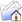LISTSERV Archives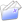SOCNET HomeSOCNET May 2007

Subject:Summary of responses to post regarding geodesic distance

From:Date:Wed, 23 May 2007 12:51:30 -0700

Content-Type:text/plain

Parts/Attachments: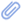text/plain (71 lines)
 ```***** To join INSNA, visit http://www.insna.org ***** Provided below is a summary of the responses I received to the following post: Original post: (to SOCNET on 5/19/07) I have computed the geodesic distances for all possible dyadic combinations of actors in a network. The network is not completely connected and thus for some dyads, there is no path between them. Theoretically, the geodesic distance between two such actors is infinity. One approach would be to drop dyadic observations with an infinite path length from my analysis and treat them as missing data. However, I am looking for suggestions about assigning an actual value for distance (or a transformation thereof) for such dyads so they do not fall out of my analysis. I would appreciate any and all help from the list. Thanks. Responses 1) ...from a mathematical point of view, the distance between disconnected nodes is actually undefined rather than infinite. But inserting infinity certainly works well enough. The other popular values are N (i.e., one more than maximum distance possible in a graph with n nodes) and D+1 (where D is the max diameter of any component). 2) One strategy is to use N+1 (where N = number of nodes in graph); this will not change the median value much, and allows you to keep the dyad in your analyses. 3) When I deal with disconnected graphs and distance, I often invert the distance, because an infinite distance then becomes 0, while a distance of 1 stays as 1 (a distance of 2 is 0.5, 3 is 1/3, etc...). 4)Something to consider: shortest path length may not be the right metric. If A and B are connected by 37 distinct paths of length 2, is that a closer association than if A and B are connected via exactly one path of length 2? 5) While analytically, I think you want to be careful about the implications of dropping infinite distances, I have found it useful for graphing and similar applications to assign an arbitrary distance of n (the number of nodes/actors), since the maximum width of a connected graph is n-1. Plotting infinity, or things like average distance, become incalculable when infinities are present. 6) In a regression framework, you could create dummy variable for various distances (e.g., geo distance<3, 3<= dist < 5, etc) and then simply create a dummy variable for "more than X," where X is the observed maximum. Then, you could include the pairs in a data set without worrying that you have falsely assumed a finite distance. The maximal distance is simply a categorical variable. Corey Phelps, PhD Asst. Professor, Management & Organization University of Washington Business School Box 353200 Seattle, WA 98195 (206) 543-6579 _____________________________________________________________________ SOCNET is a service of INSNA, the professional association for social network researchers (http://www.insna.org). To unsubscribe, send an email message to [log in to unmask] containing the line UNSUBSCRIBE SOCNET in the body of the message.```

#### Search Archives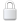Log In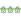Get Password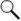Search Archives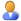Subscribe or Unsubscribe